## Waves and Sound

6-17-98

We'll shift gears again, moving on to waves.

### Types of waves

A wave is a disturbance that transfers energy from one place to another without requiring any net flow of mass. For now, we'll focus on mechanical waves, requiring a medium in which to travel. Light, and other electromagnetic waves, do not require a medium; we'll deal with those next semester.

Waves can be broadly separated into pulses and periodic waves. A pulse is a single disturbance while a periodic wave is a continually oscillating motion. There is a close connection between simple harmonic motion and periodic waves; in most periodic waves, the particles in the medium experience simple harmonic motion.

Waves can also be separated into transverse and longitudinal waves. In a transverse wave, the motion of the particles of the medium is at right angles (i.e., transverse) to the direction the wave moves. In a longitudinal wave, such as a sound wave, the particles oscillate along the direction of motion of the wave.

Surface waves, such as water waves, are generally a combination of a transverse and a longitudinal wave. The particles on the surface of the water travel in circular paths as a wave moves across the surface.

### Periodic waves

A periodic wave generally follows a sine wave pattern, as shown in the diagram.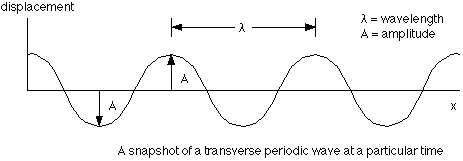A number of parameters can be defined to describe a periodic wave:

wavelength - the length of one cycle
amplitude - the maximum distance a particle gets from its undisturbed position
period (T) - time required for one cycle
frequency (f = 1/T) - number of cycles in a certain time, usually in 1 second.
speed - this is given by v = frequency x wavelengthNote that the frequency of the wave is set by whatever is producing the disturbance, while the speed is determined by the properties of the medium. The wavelength can then be found from the equation above.

A sine wave oscillation is also followed by each particle in the medium, as the following diagram shows: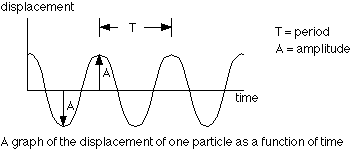There is a big difference between what the wave does and what the particles in the medium do. As the wave travels through the medium, the particles of the medium oscillate in response to the wave. In a uniform medium, the wave travels at constant speed; each particle, however, has a speed that is constantly changing. The maximum speed of a particle is determined by the wave amplitude and frequency.

### The speed of a wave

The speed of a wave traveling through a medium depends on properties of the medium. For a wave to propagate, each disturbed particle must exert a force on its neighbor. The wave speed is essentially the speed at which the neighboring particle responds to this force; that response time is determined by the mass of the particle and the size of the force exerted. For a transverse wave on a rope or stretched spring, the wave speed is determined by the tension (F) and the mass per unit length:For a sound wave, the speed is determined by either Young's modulus or the bulk modulus (discussed in chapter 10) and the density:### Reflection of waves

This applies to both pulses and periodic waves, although it's easier to see for pulses. Consider what happens when a pulse reaches the end of its rope, so to speak. The wave will be reflected back along the rope.

If the end is fixed, the pulse will be reflected upside down (also known as a 180° phase shift).

If the end is free, the pulse comes back the same way it went out (so no phase change).

If the pulse is traveling along one rope tied to another rope, of different density, some of the energy is transmitted into the second rope and some comes back. For a pulse going from a light rope to a heavy rope, the reflection occurs as if the end is fixed. From heavy to light, the reflection is as if the end is free.

### Sound waves

Anything that vibrates is producing sound; sound is simply a longitudinal wave passing through a medium via the vibration of particles in the medium.

Consider a sound wave traveling in air. This sound wave is generated by a speaker broadcasting a single frequency, so the speaker cone is oscillating back and forth in simple harmonic motion. The motion of the speaker slightly changes the pressure in the air next to it. When the speaker moves out, the air is compressed more than average and the pressure increases; when the speaker moves in, the pressure decreases slightly. These pressure changes propagate away from the speaker, and this is what a sound wave is, a pressure wave.

Sound waves travel through different media in the same manner, with the density fluctuating in response to the wave. The areas of lower pressure (or, equivalently, density) are known as rarefractions, while the areas of higher density are called compressions or condensations.

Sound waves generally involve very small fluctuations in pressure or density. In air, sound is just a tiny ripple superimposed on atmospheric pressure. A loud sound involves a pressure-wave amplitude of about one millionth of atmospheric pressure, and we can hear sounds that are of considerably smaller amplitude than that.

### The speed of sound

A sound wave propagates by alternately compressing and expanding the medium. The speed of sound thus depends on how easily a medium can be compressed (or, equivalently, expanded). Sound travels fastest in media which are hard to compress (like different metals) because if one particle moves in response to a pressure wave, its neighbor will respond quickly. In easily-compressible media, such as most gases, the speed of sound is slower because particles respond more slowly to motion of neighboring particles.

The compressibility of a material is measured by the bulk modulus, discussed in chapter 10. The speed of sound also depends on density, and is given by: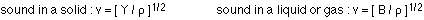For an ideal gas, it turns out that the speed of sound is given by: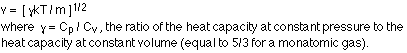m is the mass of one molecule.

In air at 0 °C, the speed of sound is 331 m/s. It increases with temperature. In liquids, the speed of sound is more like 1000 m/s, and in a typical solid more like 5000 m/s.

### Intensity

Just as the total energy in an oscillating spring is proportional to the square of the amplitude of the oscillation, the energy in a sound wave is proportional to the square of the amplitude of the pressure difference. A sound wave is usually characterized by the power (energy / second) it carries: the intensity is the power (P) divided by the area (A) the sound power passes through:The human ear can detect sound of very low intensity. The smallest detectable sound intensity, known as the threshold of hearing, is about 1 x 10^-12 W / m2. Sound that is 1 W / m2 is intense enough to damage the ear.

If sound moves away from a source uniformly in all directions, the intensity decreases the further away from the source you are. In fact, the intensity is inversely proportional to the square of the distance from the source. At a distance r away from a source sending out sound with a power P, the sound passes through a sphere with a surface area equal to. The intensity is thus:This dependence on 1/r2 applies to anything emitted uniformly in all directions (sound, light, etc.).

### Decibels

The human ear has an incredibly large range, being able to detect sound intensities from 1 x 10-12 W / m2 to 1 W / m2. A more convenient way to measure the loudness of sound is in decibels (dB); in decibels, the range of human hearing goes from 0 dB to 120 dB. The ear responds to the loudness of sound logarithmically, so the decibel scale is a logarithmic scale:On the decibel scale, doubling the intensity corresponds to an increase of 3 dB. This does not correspond to a perceived doubling of loudness, however. We perceive loudness to be doubled when the intensity increases by a factor of 10! This corresponds to a 10 dB increase. A change by 1 dB is about the smallest change a human being can detect.

### An example using decibels

A particular sound has an intensity of 1 x 10-6 W / m2 . What is this in decibels? If the intensity is increased by 15 dB, what is the new intensity in W / m2?

The intensity in dB can be found by simply applying the equation: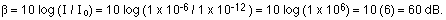If this is increased to 75 dB, the new intensity can be found like this: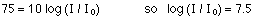Therefore, I / Io = the inverse log of 7.5. Taking the inverse log of 7.5 means simply raising 10 to the 7.5 power, so:This is 31.6 times as much as the original intensity.